You dont have javascript enabled! Please enable it!

### BECE Math 1990 – Essay

BECE: MATHS ESSAY 1990

#### B.E.C.E – 1990 ESSAY TYPE QUESTIONS

##### QUESTION 1

a) List the members of each of the sets

B = {whole numbers from 20 to 30} and D = {factors of 63}
List the members of
i) B ⋂ D
ii) B ⋃ D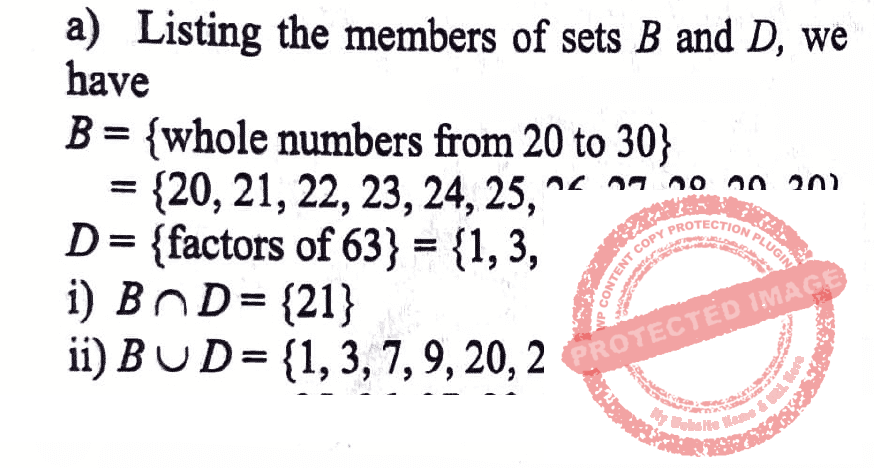b) In a class of 60 students, 46 passed Mathematics and 42 passed English language. Every student passed at least one of the two subjects.
i) Illustrate this information on a Venn diagram.
ii) How many students passed in both subjects?
Let n represent the number of students who passed in both subjects.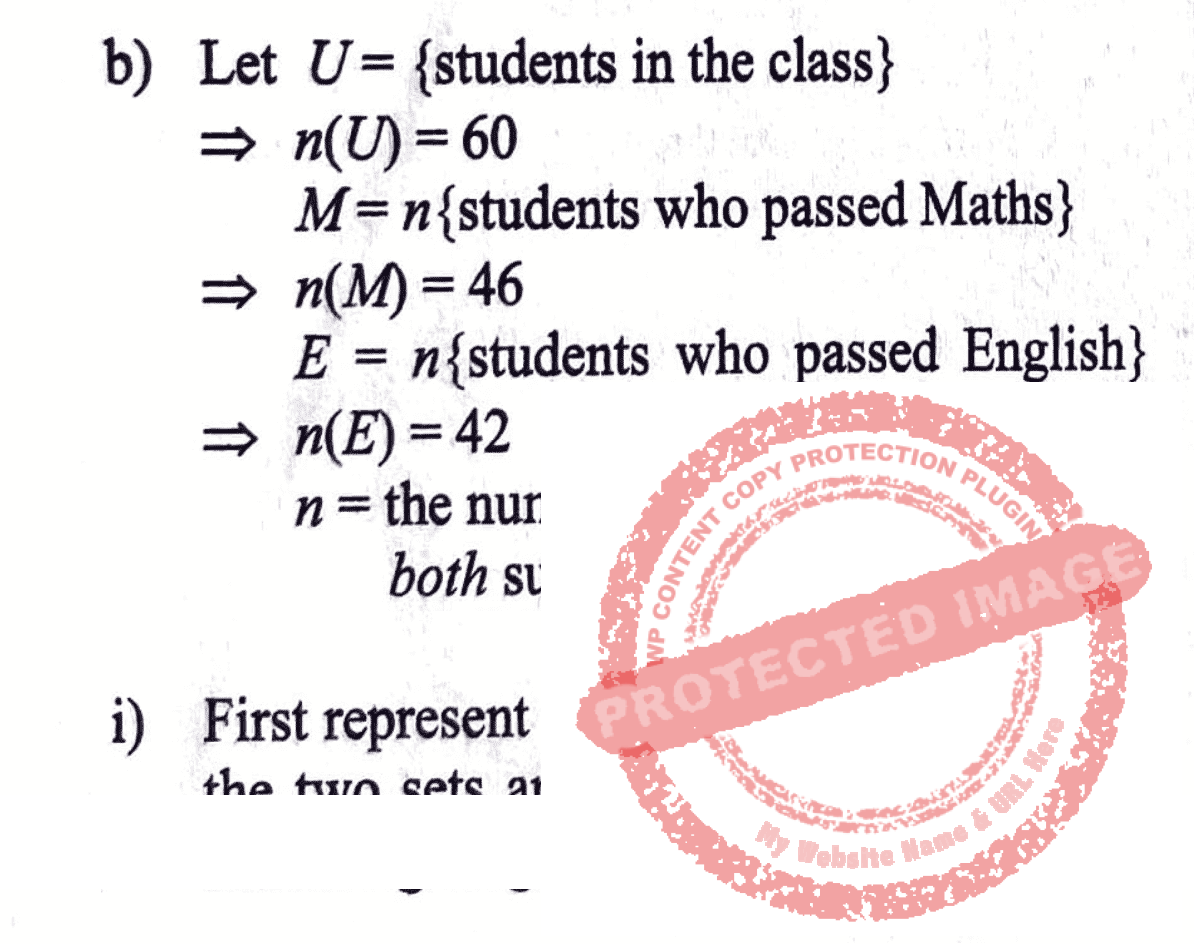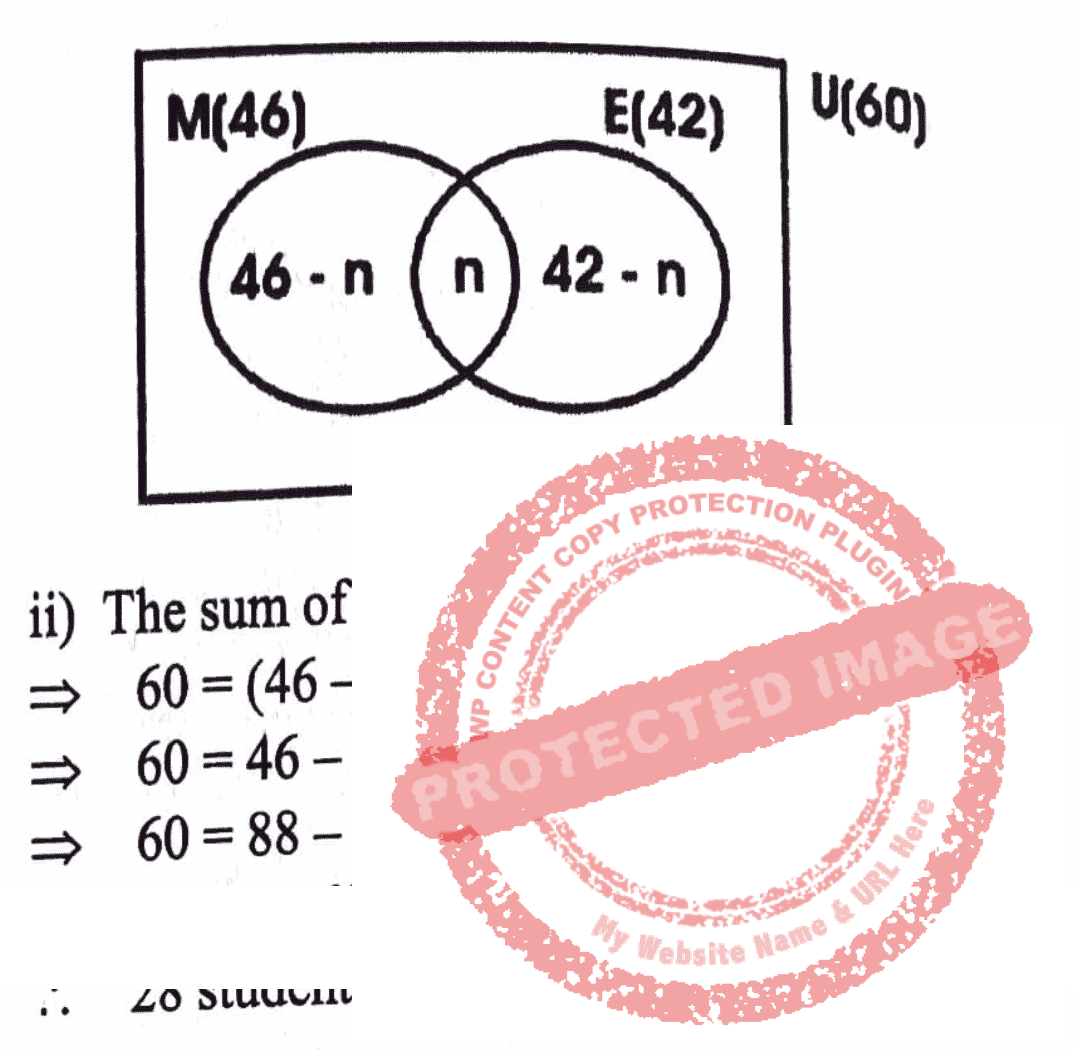QUESTION 2

a) Factorise completely
3a² + 2ab – 12ac – 8bcb) Solve x/4 + 3/5 = 3x/2 -2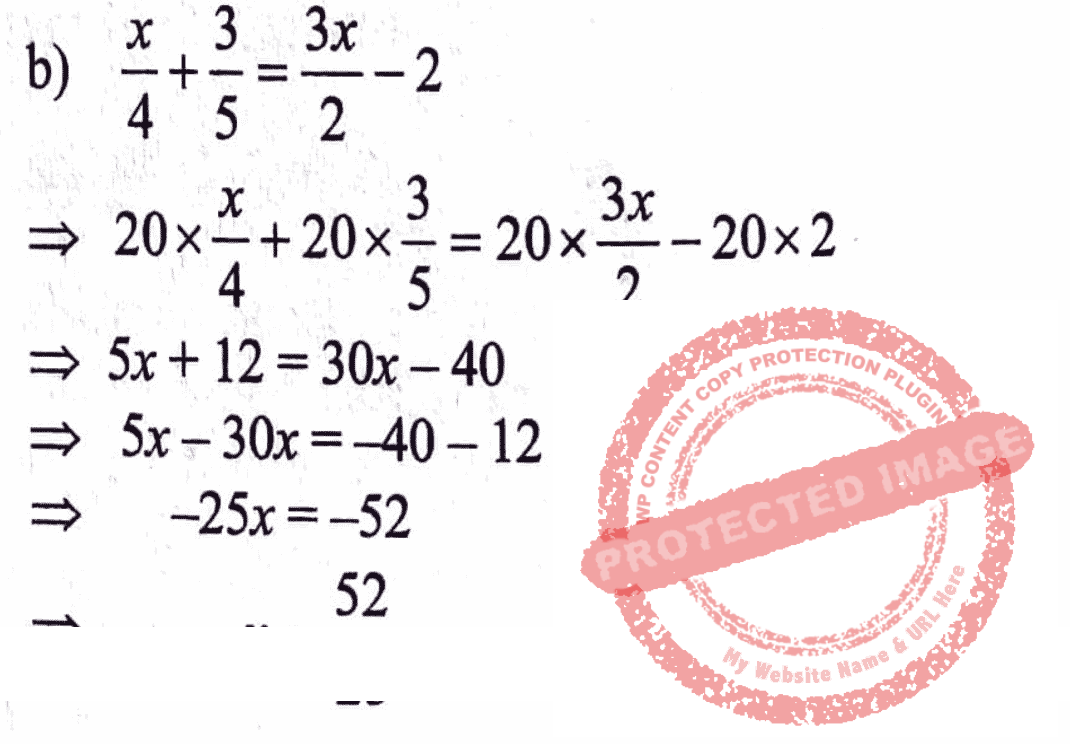c) Find the solution set of x + 3 > 19 – 3x, where x is a real number.

Illustrate answer on the number line.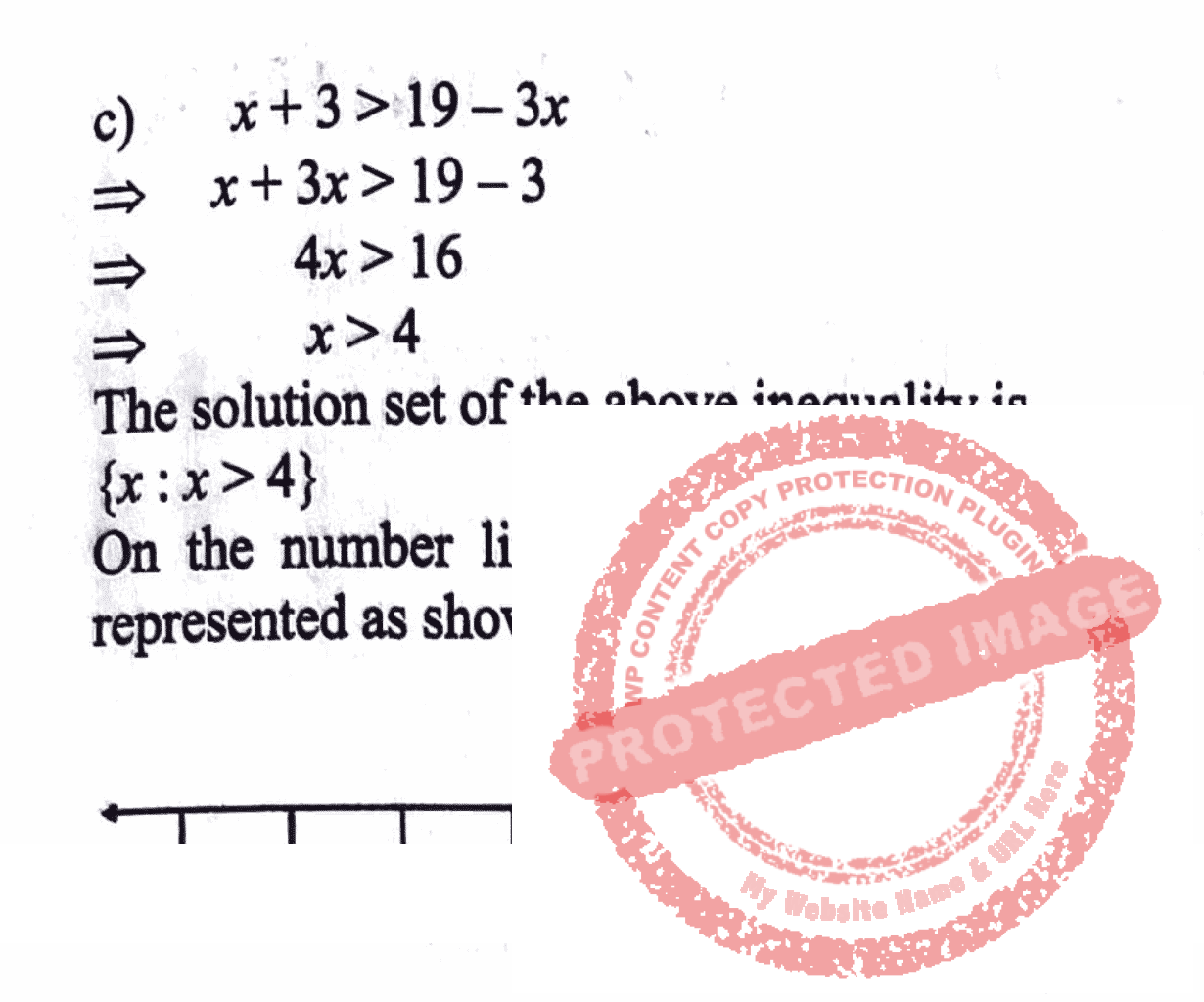QUESTION 3

a) Using a ruler and a pair of compasses only;

i) Construct a triangle PQR such that
IPQI = 6 cm, IQRI = 4 cm and angle PQR = 90°
ii) Construct perpendicular bisectors (mediators) of PQ and QR. Name the intersection of the mediators O.
iii) Draw a circle with O as centre and OQ as radius.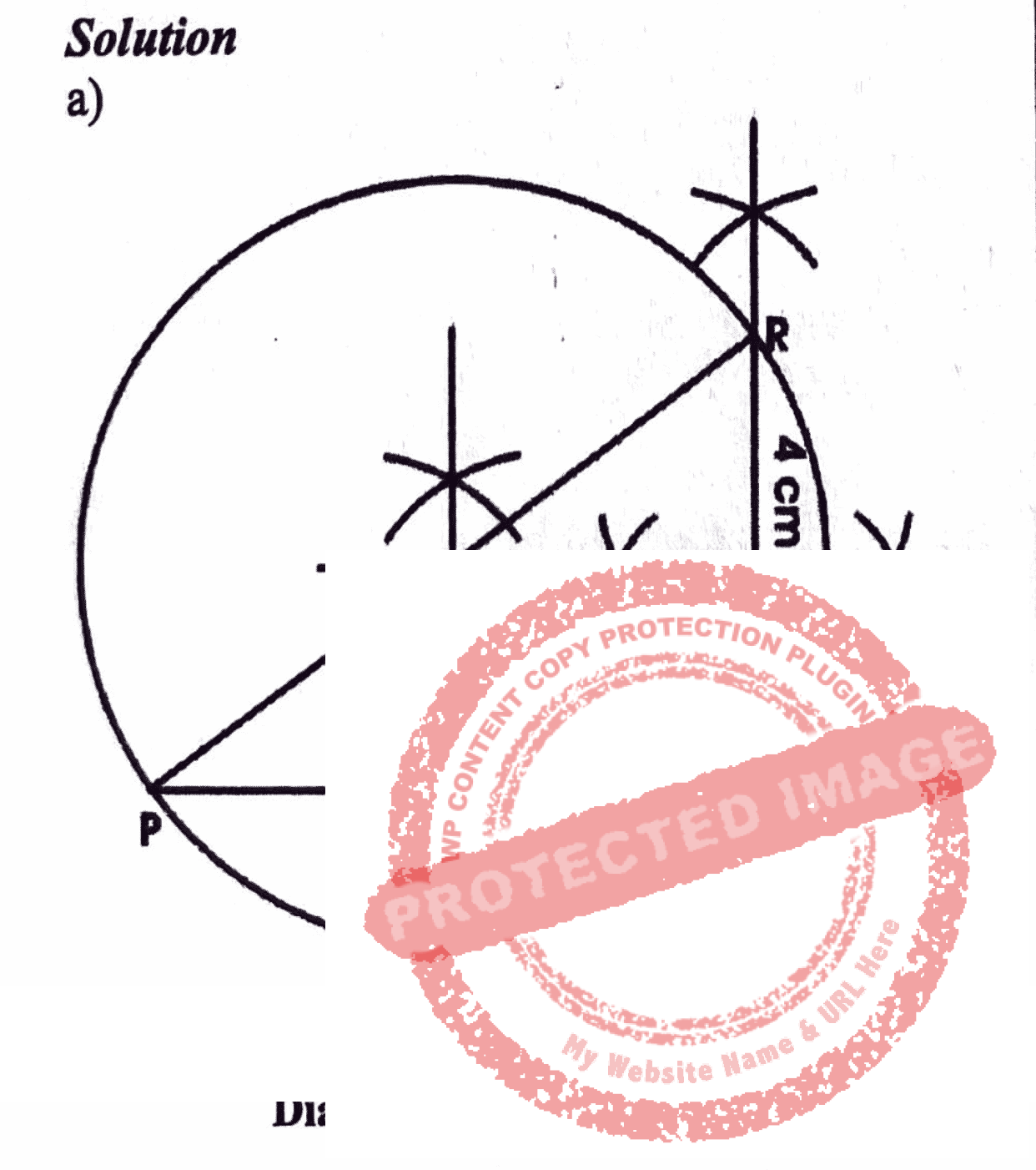b) Measure
i) IPRI
ii) angle QPR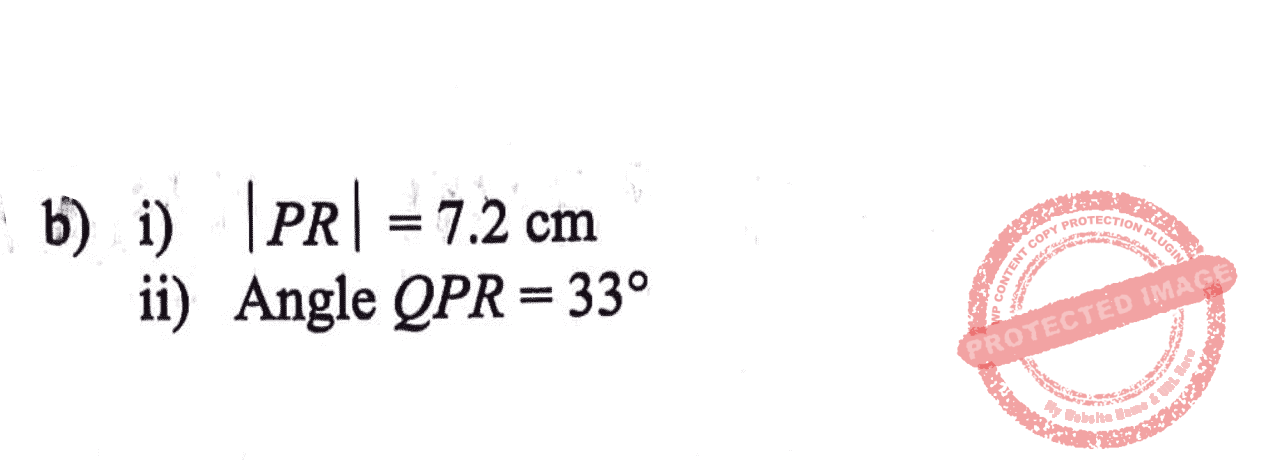QUESTION 4

The following is the result of a survey conducted in a class of a Junior Secondary School to find the favourite soft drink of each pupil in the class.

a) Draw a bar chart showing this information, using a scale of 2cm to 1 unit on the vertical axis.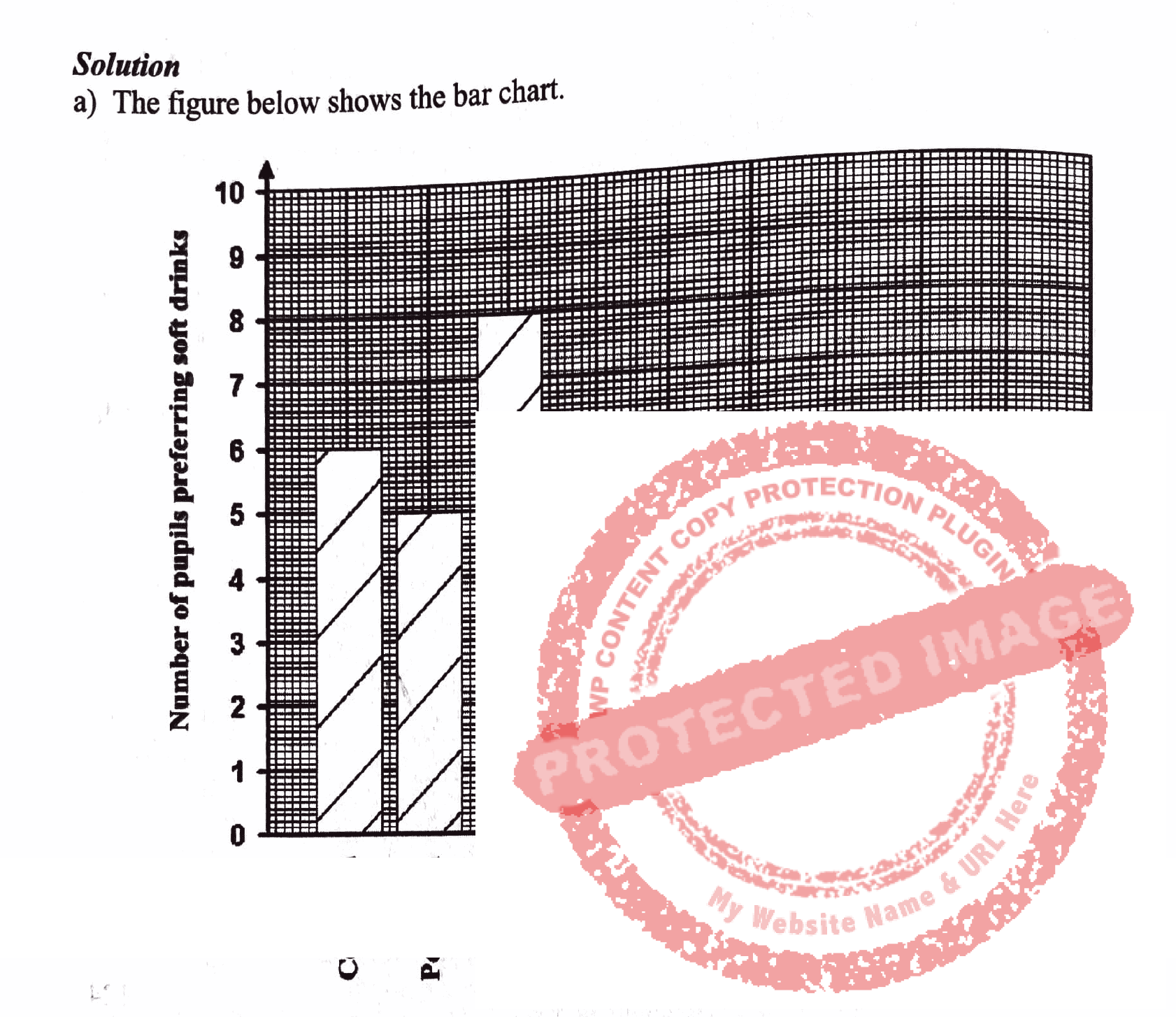b) How many pupils are in the class?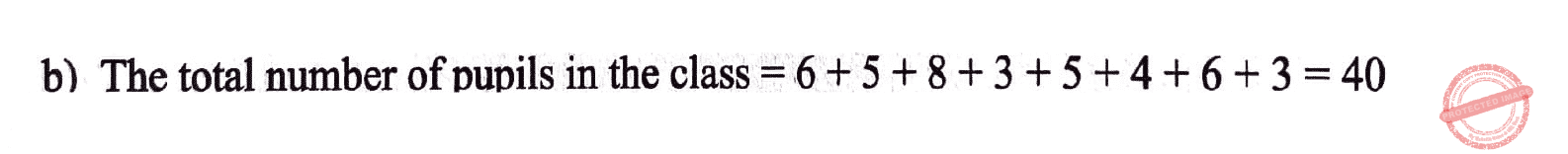c) What percentage of pupils in the class prefer Club Cola?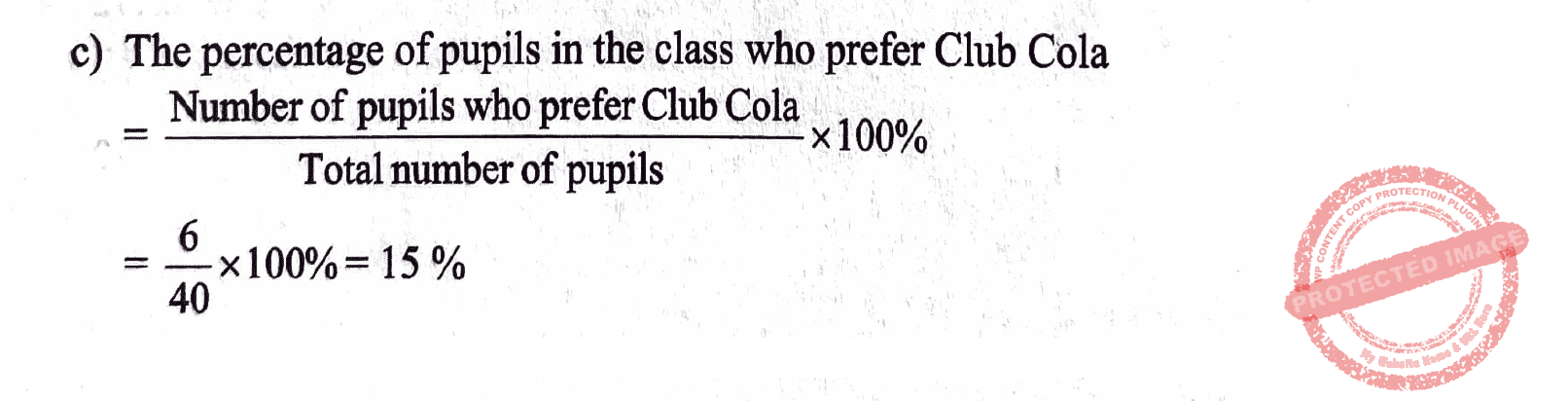error: